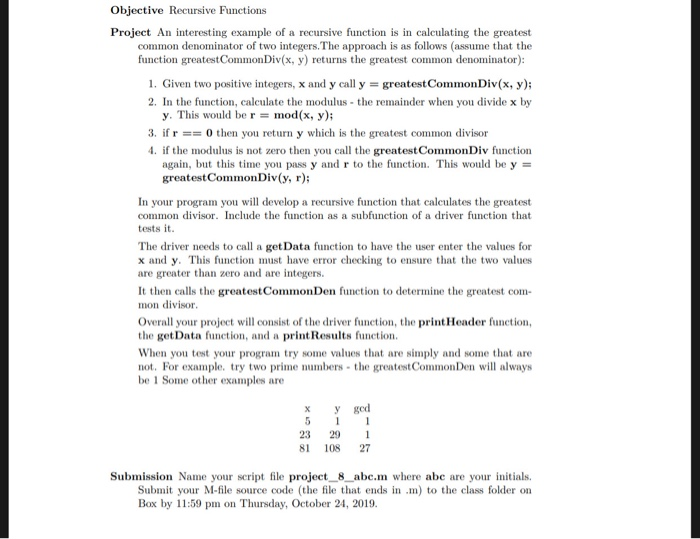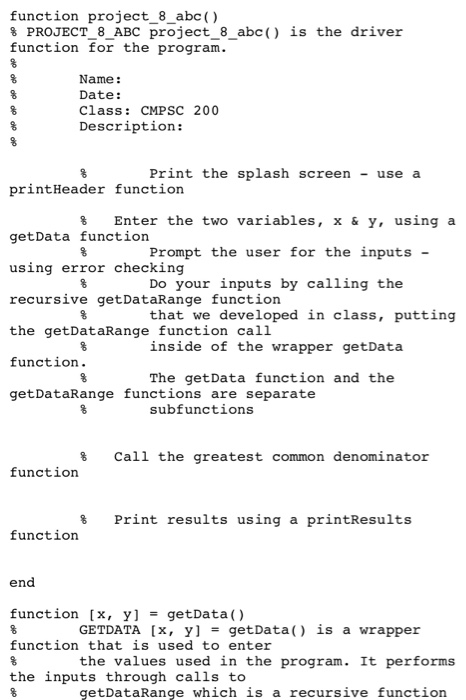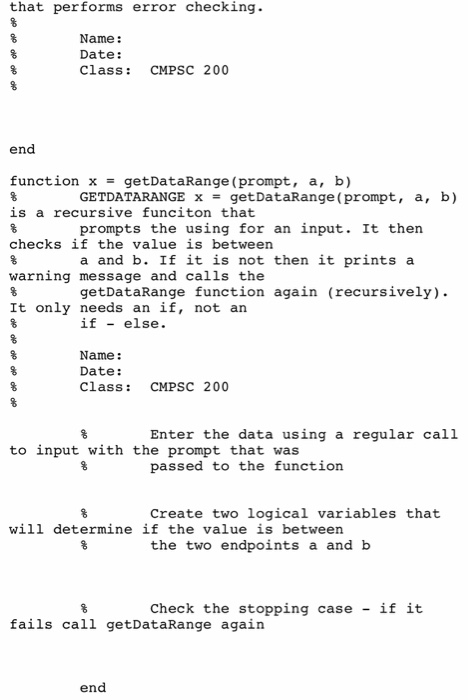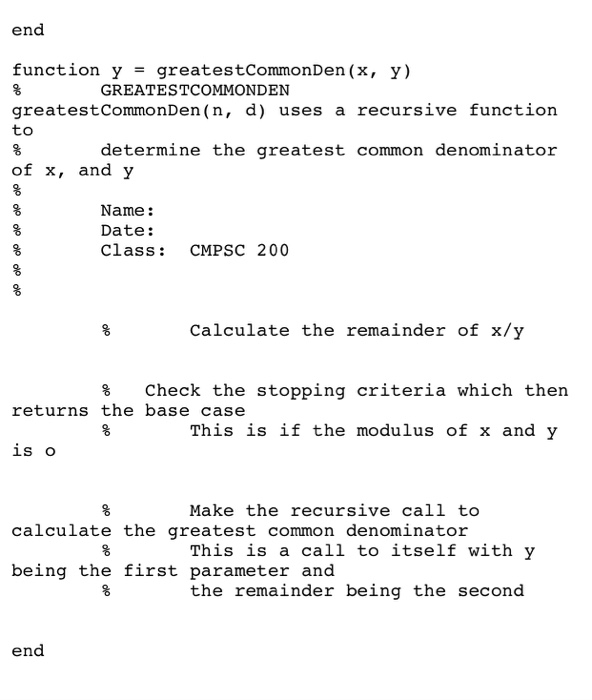# (Solved) : Objective Recursive Functions Project Interesting Example Recursive Function Calculating G Q41353458 . . .Objective Recursive Functions Project An interesting example of a recursive function is in calculating the greatest common denominator of two integers. The approach is as follows assume that the function greatestCommon Div(x, y) returns the greatest common denominator): 1. Given two positive integers, x and y call y = greatestCommon Div(x, y); 2. In the function, calculate the modulus – the remainder when you divide x by y. This would be r = mod(x, y); 3. if r == 0 then you return y which is the greatest common divisor 4. if the modulus is not zero then you call the greatestCommon Div function again, but this time you pass y and r to the function. This would be y = greatestCommon Div(y, r); In your program you will develop a recursive function that calculates the greatest common divisor. Include the function as a subfunction of a driver function that tests it. The driver needs to call a get Data function to have the user enter the values for x and y. This function must have error checking to ensure that the two values are greater than zero and are integers. It then calls the greatestCommonDen function to determine the greatest com- mon divisor Overall your project will consist of the driver function, the print Header function, the getData function, and a print Results function. When you test your program try some values that are simply and some that are not. For example, try two prime numbers – the greatestCommon Den will always be 1 Some other examples are X y ged 23 81 29 108 1 27 Submission Name your script file project_8_abc.m where abc are your initials. Submit your M-file source code (the file that ends in .m) to the class folder on Box by 11:59 pm on Thursday, October 24, 2019. function project_8_abc() 8 PROJECT_8_ABC project_8_abc() is the driver function for the program. Name: Date: Class: CMPSC 200 Description: Print the splash screen – use a print Header function & Enter the two variables, X & y, using a get Data function Prompt the user for the inputs – using error checking \$ Do your inputs by calling the recursive getDataRange function that we developed in class, putting the getDataRange function call inside of the wrapper getData function. The getData function and the getDataRange functions are separate % Subfunctions call the greatest common denominator 8 function Print results using a printResults % function end function (x, y) = getData() GETDATA (x, y) = getData() is a wrapper function that is used to enter the values used in the program. It performs the inputs through calls to getDataRange which is a recursive function that performs error checking. Name: Date: Class: CMPSC 200 end function x = getDataRange (prompt, a, b) % GETDATARANGE x = getDataRange (prompt, a, b) is a recursive funciton that prompts the using for an input. It then checks if the value is between a and b. If it is not then it prints a warning message and calls the getDataRange function again (recursively). It only needs an if, not an if – else. Name : Date: CMPSC 200 Enter the data using a regular call to input with the prompt that was passed to the function Create two logical variables that will determine if the value is between the two endpoints a and b Check the stopping case – if it fails call getDataRange again end end end function y = greatestCommonDen(x, y) % GREATESTCOMMONDEN greatestCommonDen(n, d) uses a recursive function to determine the greatest common denominator of x, and y Name: Date: Class: CMPSC 200 Calculate the remainder of x/y % Check the stopping criteria which then returns the base case This is if the modulus of x and y is o Make the recursive call to calculate the greatest common denominator This is a call to itself with y being the first parameter and the remainder being the second end Show transcribed image text Objective Recursive Functions Project An interesting example of a recursive function is in calculating the greatest common denominator of two integers. The approach is as follows assume that the function greatestCommon Div(x, y) returns the greatest common denominator): 1. Given two positive integers, x and y call y = greatestCommon Div(x, y); 2. In the function, calculate the modulus – the remainder when you divide x by y. This would be r = mod(x, y); 3. if r == 0 then you return y which is the greatest common divisor 4. if the modulus is not zero then you call the greatestCommon Div function again, but this time you pass y and r to the function. This would be y = greatestCommon Div(y, r); In your program you will develop a recursive function that calculates the greatest common divisor. Include the function as a subfunction of a driver function that tests it. The driver needs to call a get Data function to have the user enter the values for x and y. This function must have error checking to ensure that the two values are greater than zero and are integers. It then calls the greatestCommonDen function to determine the greatest com- mon divisor Overall your project will consist of the driver function, the print Header function, the getData function, and a print Results function. When you test your program try some values that are simply and some that are not. For example, try two prime numbers – the greatestCommon Den will always be 1 Some other examples are X y ged 23 81 29 108 1 27 Submission Name your script file project_8_abc.m where abc are your initials. Submit your M-file source code (the file that ends in .m) to the class folder on Box by 11:59 pm on Thursday, October 24, 2019.
function project_8_abc() 8 PROJECT_8_ABC project_8_abc() is the driver function for the program. Name: Date: Class: CMPSC 200 Description: Print the splash screen – use a print Header function & Enter the two variables, X & y, using a get Data function Prompt the user for the inputs – using error checking \$ Do your inputs by calling the recursive getDataRange function that we developed in class, putting the getDataRange function call inside of the wrapper getData function. The getData function and the getDataRange functions are separate % Subfunctions call the greatest common denominator 8 function Print results using a printResults % function end function (x, y) = getData() GETDATA (x, y) = getData() is a wrapper function that is used to enter the values used in the program. It performs the inputs through calls to getDataRange which is a recursive function
that performs error checking. Name: Date: Class: CMPSC 200 end function x = getDataRange (prompt, a, b) % GETDATARANGE x = getDataRange (prompt, a, b) is a recursive funciton that prompts the using for an input. It then checks if the value is between a and b. If it is not then it prints a warning message and calls the getDataRange function again (recursively). It only needs an if, not an if – else. Name : Date: CMPSC 200 Enter the data using a regular call to input with the prompt that was passed to the function Create two logical variables that will determine if the value is between the two endpoints a and b Check the stopping case – if it fails call getDataRange again end end
end function y = greatestCommonDen(x, y) % GREATESTCOMMONDEN greatestCommonDen(n, d) uses a recursive function to determine the greatest common denominator of x, and y Name: Date: Class: CMPSC 200 Calculate the remainder of x/y % Check the stopping criteria which then returns the base case This is if the modulus of x and y is o Make the recursive call to calculate the greatest common denominator This is a call to itself with y being the first parameter and the remainder being the second end